Publicité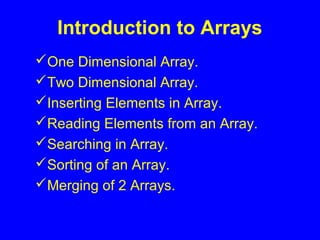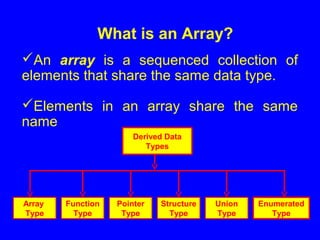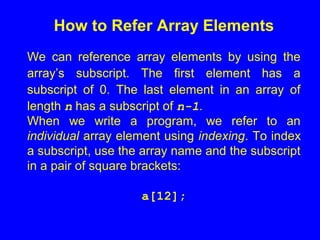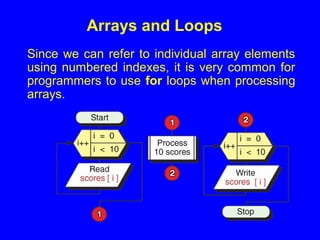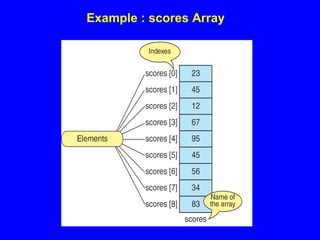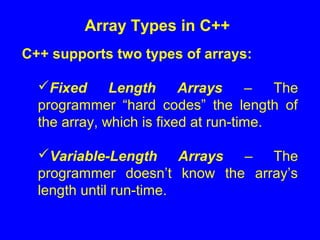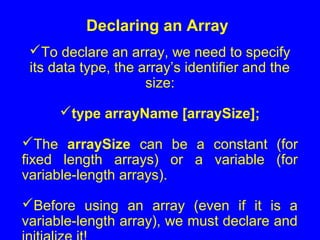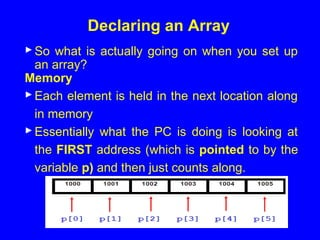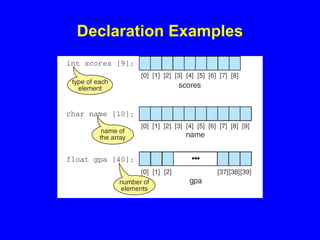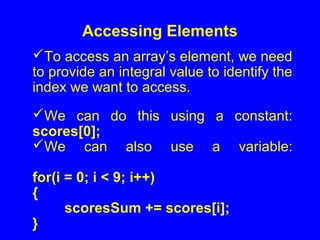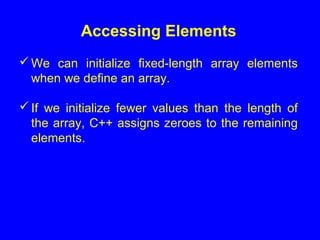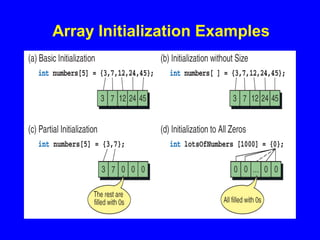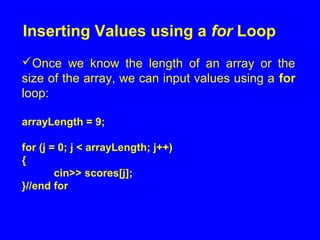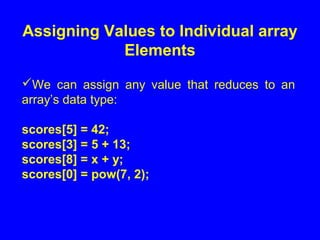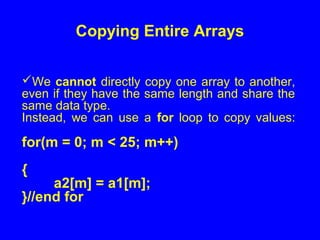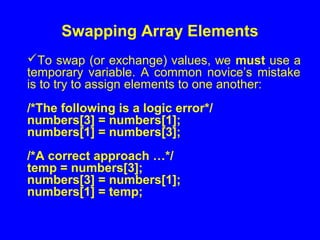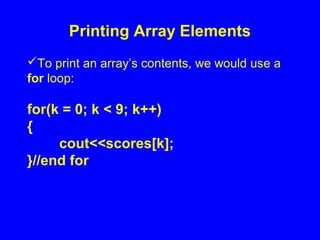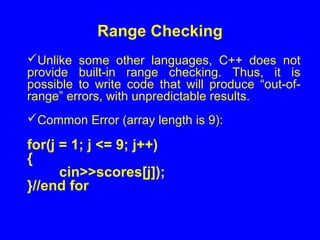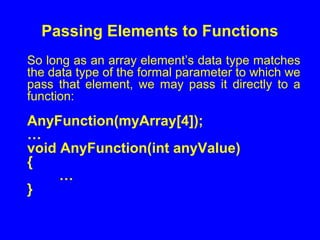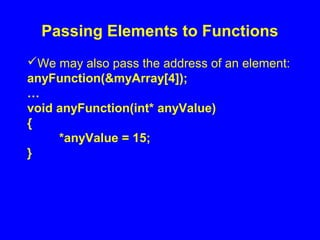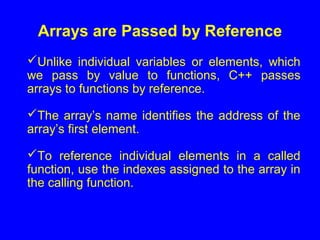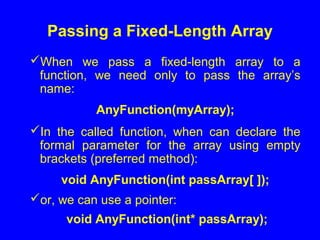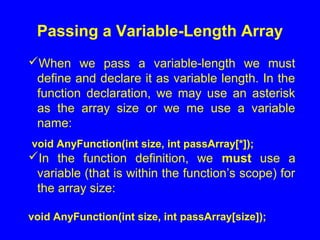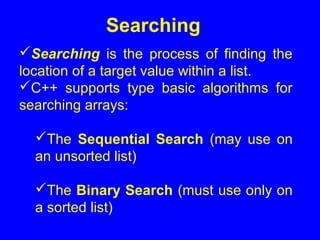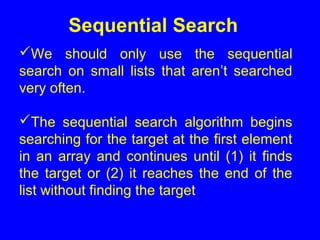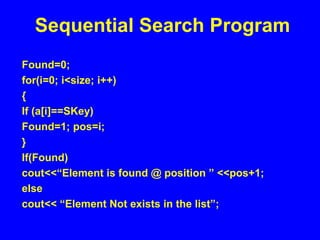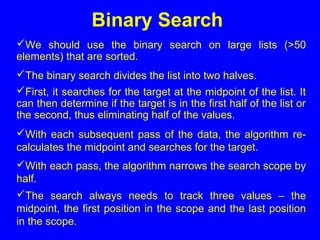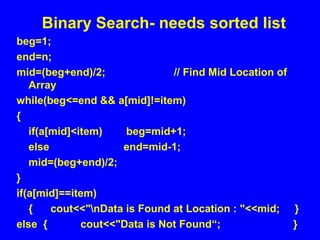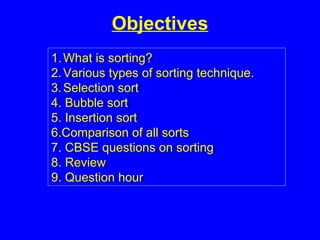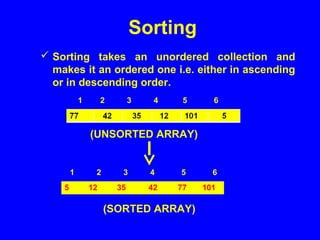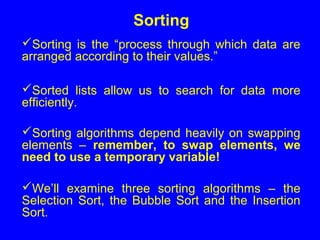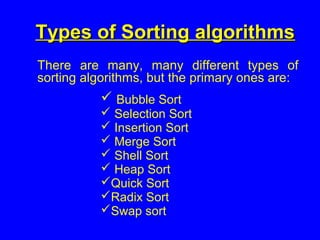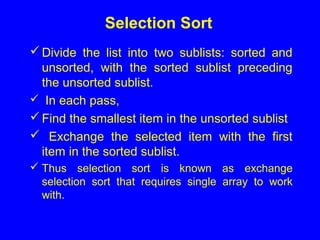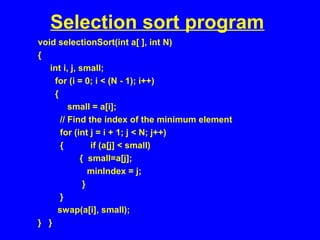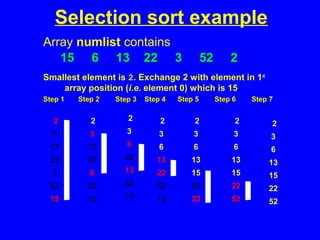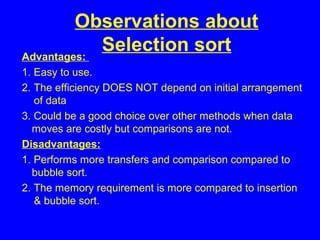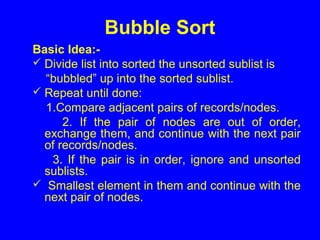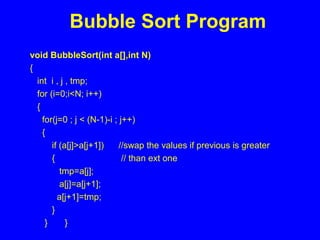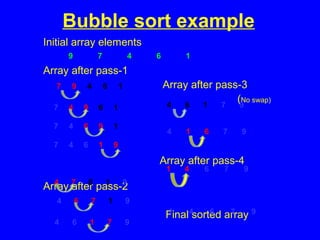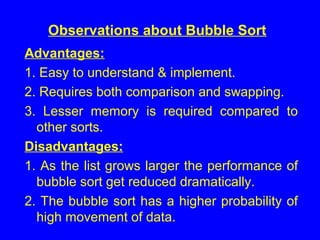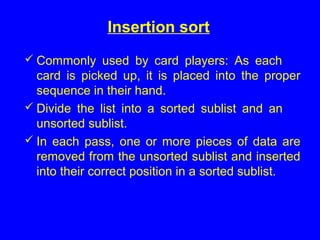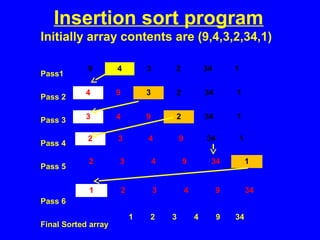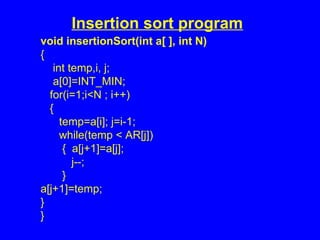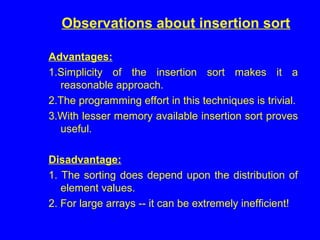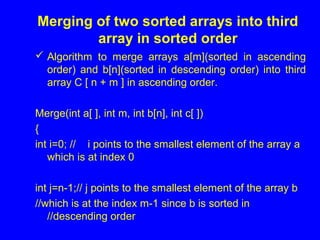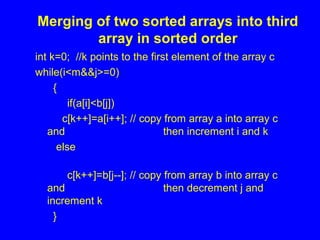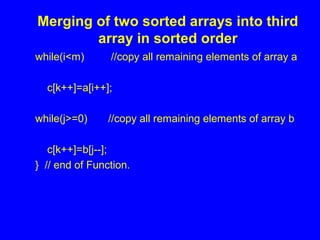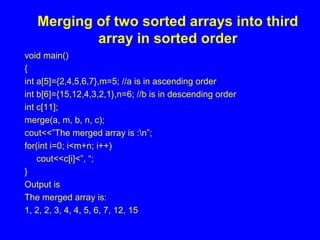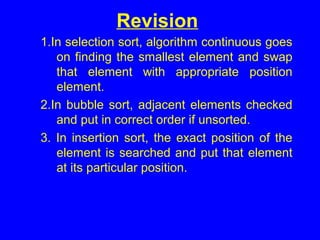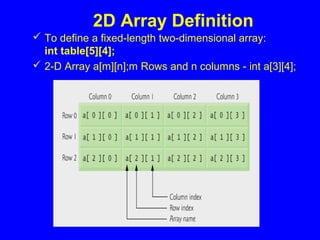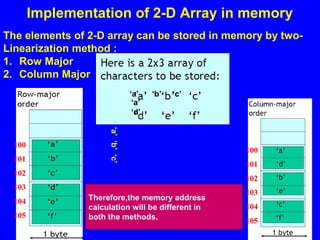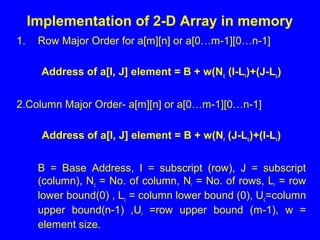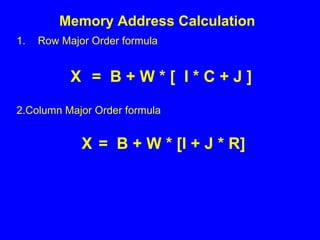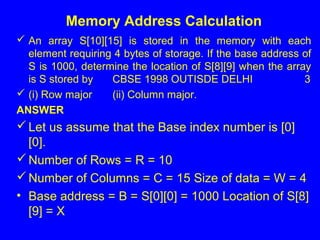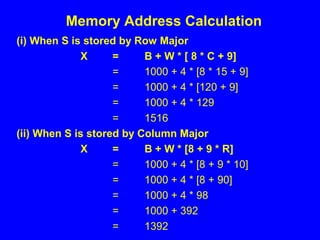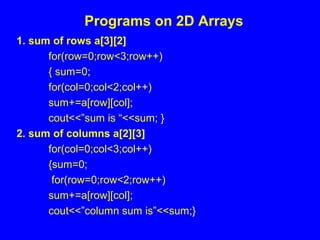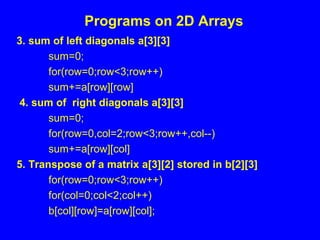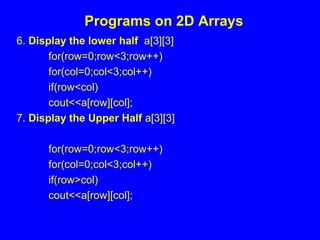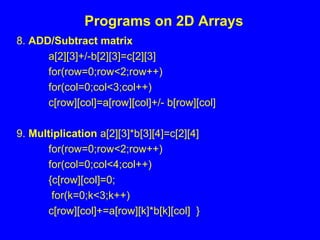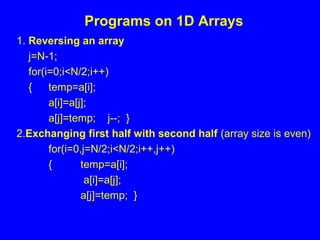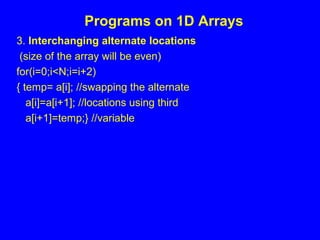1 sur 68
Publicité

### 9 Arrays

1. WEL COME PRAVEEN M JIGAJINNI PGT (Computer Science) MTech[IT],MPhil (Comp.Sci), MCA, MSc[IT], PGDCA, ADCA, Dc. Sc. & Engg. email: praveenkumarjigajinni@yahoo.co.in
2. One Dimensional Array. Two Dimensional Array. Inserting Elements in Array. Reading Elements from an Array. Searching in Array. Sorting of an Array. Merging of 2 Arrays. Introduction to Arrays
3. What is an Array? An array is a sequenced collection of elements that share the same data type. Elements in an array share the same name Enumerated Type Derived Data Types Function Type Array Type Pointer Type Structure Type Union Type
4. How to Refer Array Elements We can reference array elements by using the array’s subscript. The first element has a subscript of 0. The last element in an array of length n has a subscript of n-1. When we write a program, we refer to an individual array element using indexing. To index a subscript, use the array name and the subscript in a pair of square brackets: a;
5. Arrays and Loops Since we can refer to individual array elements using numbered indexes, it is very common for programmers to use for loops when processing arrays.
6. Example : scores Array
7. Array Types in C++ C++ supports two types of arrays: Fixed Length Arrays – The programmer “hard codes” the length of the array, which is fixed at run-time. Variable-Length Arrays – The programmer doesn’t know the array’s length until run-time.
8. Declaring an Array To declare an array, we need to specify its data type, the array’s identifier and the size: type arrayName [arraySize]; The arraySize can be a constant (for fixed length arrays) or a variable (for variable-length arrays). Before using an array (even if it is a variable-length array), we must declare and
9. Declaring an Array So what is actually going on when you set up an array? Memory Each element is held in the next location along in memory Essentially what the PC is doing is looking at the FIRST address (which is pointed to by the variable p) and then just counts along.
10. Declaration Examples
11. Accessing Elements To access an array’s element, we need to provide an integral value to identify the index we want to access. We can do this using a constant: scores; We can also use a variable: for(i = 0; i < 9; i++) { scoresSum += scores[i]; }
12. Accessing Elements We can initialize fixed-length array elements when we define an array. If we initialize fewer values than the length of the array, C++ assigns zeroes to the remaining elements.
13. Array Initialization Examples
14. Inserting Values using a for Loop Once we know the length of an array or the size of the array, we can input values using a for loop: arrayLength = 9; for (j = 0; j < arrayLength; j++) { cin>> scores[j]; }//end for
15. Assigning Values to Individual array Elements We can assign any value that reduces to an array’s data type: scores = 42; scores = 5 + 13; scores = x + y; scores = pow(7, 2);
16. Copying Entire Arrays We cannot directly copy one array to another, even if they have the same length and share the same data type. Instead, we can use a for loop to copy values: for(m = 0; m < 25; m++) { a2[m] = a1[m]; }//end for
17. Swapping Array Elements To swap (or exchange) values, we must use a temporary variable. A common novice’s mistake is to try to assign elements to one another: /*The following is a logic error*/ numbers = numbers; numbers = numbers; /*A correct approach …*/ temp = numbers; numbers = numbers; numbers = temp;
18. Printing Array Elements To print an array’s contents, we would use a for loop: for(k = 0; k < 9; k++) { cout<<scores[k]; }//end for
19. Range Checking Unlike some other languages, C++ does not provide built-in range checking. Thus, it is possible to write code that will produce “out-of- range” errors, with unpredictable results. Common Error (array length is 9): for(j = 1; j <= 9; j++) { cin>>scores[j]); }//end for
20. Passing Elements to Functions So long as an array element’s data type matches the data type of the formal parameter to which we pass that element, we may pass it directly to a function: AnyFunction(myArray); … void AnyFunction(int anyValue) { … }
21. Passing Elements to Functions We may also pass the address of an element: anyFunction(&myArray); … void anyFunction(int* anyValue) { *anyValue = 15; }
22. Arrays are Passed by Reference Unlike individual variables or elements, which we pass by value to functions, C++ passes arrays to functions by reference. The array’s name identifies the address of the array’s first element. To reference individual elements in a called function, use the indexes assigned to the array in the calling function.
23. Passing a Fixed-Length Array When we pass a fixed-length array to a function, we need only to pass the array’s name: AnyFunction(myArray); In the called function, when can declare the formal parameter for the array using empty brackets (preferred method): void AnyFunction(int passArray[ ]); or, we can use a pointer: void AnyFunction(int* passArray);
24. Passing a Variable-Length Array When we pass a variable-length we must define and declare it as variable length. In the function declaration, we may use an asterisk as the array size or we me use a variable name: void AnyFunction(int size, int passArray[*]); In the function definition, we must use a variable (that is within the function’s scope) for the array size: void AnyFunction(int size, int passArray[size]);
25. Searching & Sorting Techniques
26. Searching is the process of finding the location of a target value within a list. C++ supports type basic algorithms for searching arrays: The Sequential Search (may use on an unsorted list) The Binary Search (must use only on a sorted list) Searching
27. We should only use the sequential search on small lists that aren’t searched very often. The sequential search algorithm begins searching for the target at the first element in an array and continues until (1) it finds the target or (2) it reaches the end of the list without finding the target Sequential Search
28. Sequential Search Program Found=0; for(i=0; i<size; i++) { If (a[i]==SKey) Found=1; pos=i; } If(Found) cout<<“Element is found @ position ” <<pos+1; else cout<< “Element Not exists in the list”;
29. Binary Search We should use the binary search on large lists (>50 elements) that are sorted. The binary search divides the list into two halves. First, it searches for the target at the midpoint of the list. It can then determine if the target is in the first half of the list or the second, thus eliminating half of the values. With each subsequent pass of the data, the algorithm re- calculates the midpoint and searches for the target. With each pass, the algorithm narrows the search scope by half. The search always needs to track three values – the midpoint, the first position in the scope and the last position in the scope.
30. Binary Search- needs sorted list beg=1; end=n; mid=(beg+end)/2; // Find Mid Location of Array while(beg<=end && a[mid]!=item) { if(a[mid]<item) beg=mid+1; else end=mid-1; mid=(beg+end)/2; } if(a[mid]==item) { cout<<"nData is Found at Location : "<<mid; } else { cout<<"Data is Not Found“; }
31. Sorting Techniques
32. Objectives 1.What is sorting? 2.Various types of sorting technique. 3.Selection sort 4. Bubble sort 5. Insertion sort 6.Comparison of all sorts 7. CBSE questions on sorting 8. Review 9. Question hour 1.What is sorting? 2.Various types of sorting technique. 3.Selection sort 4. Bubble sort 5. Insertion sort 6.Comparison of all sorts 7. CBSE questions on sorting 8. Review 9. Question hour
33.  Sorting takes an unordered collection and makes it an ordered one i.e. either in ascending or in descending order. 5 12 35 42 77 101 1 2 3 4 5 6 1 2 3 4 5 6 (UNSORTED ARRAY) (SORTED ARRAY) Sorting
34. Sorting Sorting is the “process through which data are arranged according to their values.” Sorted lists allow us to search for data more efficiently. Sorting algorithms depend heavily on swapping elements – remember, to swap elements, we need to use a temporary variable! We’ll examine three sorting algorithms – the Selection Sort, the Bubble Sort and the Insertion Sort.
35. Types of Sorting algorithmsTypes of Sorting algorithms There are many, many different types of sorting algorithms, but the primary ones are:  Bubble Sort  Selection Sort  Insertion Sort  Merge Sort  Shell Sort  Heap Sort Quick Sort Radix Sort Swap sort
36. Divide the list into two sublists: sorted and unsorted, with the sorted sublist preceding the unsorted sublist.  In each pass, Find the smallest item in the unsorted sublist  Exchange the selected item with the first item in the sorted sublist.  Thus selection sort is known as exchange selection sort that requires single array to work with. Selection Sort
37. Selection sort program void selectionSort(int a[ ], int N) { int i, j, small; for (i = 0; i < (N - 1); i++) { small = a[i]; // Find the index of the minimum element for (int j = i + 1; j < N; j++) { if (a[j] < small) { small=a[j]; minIndex = j; } } swap(a[i], small); } }
38. Selection sort example Array numlist contains Smallest element is 2. Exchange 2 with element in 1st array position (i.e. element 0) which is 15 Step 1Step 1 Step 2 Step 3 Step 4 Step 5 Step 6 Step 7Step 2 Step 3 Step 4 Step 5 Step 6 Step 7 15 6 13 22 3 52 2 2 6 13 22 3 52 15 2 3 13 22 6 52 15 2 3 6 22 13 52 15 2 3 6 13 22 52 15 2 3 6 13 15 52 22 2 3 6 13 15 22 52 2 3 6 13 15 22 52
39. Observations about Selection sortAdvantages: 1. Easy to use. 2. The efficiency DOES NOT depend on initial arrangement of data 3. Could be a good choice over other methods when data moves are costly but comparisons are not. Disadvantages: 1. Performs more transfers and comparison compared to bubble sort. 2. The memory requirement is more compared to insertion & bubble sort.
40. Basic Idea:-  Divide list into sorted the unsorted sublist is “bubbled” up into the sorted sublist.  Repeat until done: 1.Compare adjacent pairs of records/nodes. 2. If the pair of nodes are out of order, exchange them, and continue with the next pair of records/nodes. 3. If the pair is in order, ignore and unsorted sublists.  Smallest element in them and continue with the next pair of nodes. Bubble Sort
41. void BubbleSort(int a[],int N) { int i , j , tmp; for (i=0;i<N; i++) { for(j=0 ; j < (N-1)-i ; j++) { if (a[j]>a[j+1]) //swap the values if previous is greater { // than ext one tmp=a[j]; a[j]=a[j+1]; a[j+1]=tmp; } } } Bubble Sort Program
42. Bubble sort example Initial array elements Array after pass-1 Array after pass-3 (No swap) Array after pass-4 Array after pass-2 Final sorted array 9 7 4 6 1 7 9 4 6 1 7 4 9 6 1 7 4 6 9 1 4 7 6 1 9 7 4 6 1 9 4 6 7 1 9 4 6 1 7 9 4 1 6 7 9 1 4 6 7 9 1 4 6 7 9 4 6 1 7 9
43. Observations about Bubble Sort Advantages: 1. Easy to understand & implement. 2. Requires both comparison and swapping. 3. Lesser memory is required compared to other sorts. Disadvantages: 1. As the list grows larger the performance of bubble sort get reduced dramatically. 2. The bubble sort has a higher probability of high movement of data.
44. Insertion sort  Commonly used by card players: As each card is picked up, it is placed into the proper sequence in their hand.  Divide the list into a sorted sublist and an unsorted sublist.  In each pass, one or more pieces of data are removed from the unsorted sublist and inserted into their correct position in a sorted sublist.
45. Insertion sort program Initially array contents are (9,4,3,2,34,1) Pass1 Pass 2 Pass 3 Pass 4 Pass 5 Pass 6 Final Sorted array 9 4 3 2 34 1 4 9 3 2 34 1 3 4 9 2 34 1 2 3 4 9 34 1 2 3 4 9 34 1 1 2 3 4 9 34 1 2 3 4 9 34
46. Insertion sort program void insertionSort(int a[ ], int N) { int temp,i, j; a=INT_MIN; for(i=1;i<N ; i++) { temp=a[i]; j=i-1; while(temp < AR[j]) { a[j+1]=a[j]; j--; } a[j+1]=temp; } }
47. Observations about insertion sort Advantages: 1.Simplicity of the insertion sort makes it a reasonable approach. 2.The programming effort in this techniques is trivial. 3.With lesser memory available insertion sort proves useful. Disadvantage: 1. The sorting does depend upon the distribution of element values. 2. For large arrays -- it can be extremely inefficient!
48. Merging of two sorted arrays into third array in sorted order  Algorithm to merge arrays a[m](sorted in ascending order) and b[n](sorted in descending order) into third array C [ n + m ] in ascending order. Merge(int a[ ], int m, int b[n], int c[ ]) { int i=0; // i points to the smallest element of the array a which is at index 0 int j=n-1;// j points to the smallest element of the array b //which is at the index m-1 since b is sorted in //descending order
49. Merging of two sorted arrays into third array in sorted order int k=0; //k points to the first element of the array c while(i<m&&j>=0) { if(a[i]<b[j]) c[k++]=a[i++]; // copy from array a into array c and then increment i and k else c[k++]=b[j--]; // copy from array b into array c and then decrement j and increment k }
50. Merging of two sorted arrays into third array in sorted order while(i<m) //copy all remaining elements of array a c[k++]=a[i++]; while(j>=0) //copy all remaining elements of array b c[k++]=b[j--]; } // end of Function.
51. Merging of two sorted arrays into third array in sorted order void main() { int a={2,4,5,6,7},m=5; //a is in ascending order int b={15,12,4,3,2,1},n=6; //b is in descending order int c; merge(a, m, b, n, c); cout<<”The merged array is :n”; for(int i=0; i<m+n; i++) cout<<c[i]<”, “; } Output is The merged array is: 1, 2, 2, 3, 4, 4, 5, 6, 7, 12, 15
52. CBSE Questions on Sorting 1.Consider the following array of integers 42,29,74,11,65,58, use insertion sort to sort them in ascending order and indicate the sequence of steps required. 2. The following array of integers is to arranged in ascending order using the bubble sort technique : 26,21,20,23,29,17. Give the contents of array after each iteration. 3. Write a C++ function to arrange the following Employee structure in descending order of their salary using bubble sort. The array and its size is required to be passed as parameter to the function. Definition of Employee structure struct Employee { int Eno; char Name; float salary; };
53. Revision 1.In selection sort, algorithm continuous goes on finding the smallest element and swap that element with appropriate position element. 2.In bubble sort, adjacent elements checked and put in correct order if unsorted. 3. In insertion sort, the exact position of the element is searched and put that element at its particular position.
54. 2D Array Definition  To define a fixed-length two-dimensional array: int table;  2-D Array a[m][n];m Rows and n columns - int a;
55. 55 Implementation of 2-D Array in memory The elements of 2-D array can be stored in memory by two- Linearization method : 1. Row Major 2. Column Major 100 101 102 103 104 105 100 101 102 103 104 105 ‘a’ ‘b’ ‘c’ ‘a’ ‘d’‘a’‘b’‘c’ What is the address of ‘c’ in row major ? Ans - 102 What is the address of ‘c’ in column major ? Ans - 104 Therefore,the memory address calculation will be different in both the methods.
56. 1. Row Major Order for a[m][n] or a[0…m-1][0…n-1] Address of a[I, J] element = B + w(Nc (I-Lr)+(J-Lc) 2.Column Major Order- a[m][n] or a[0…m-1][0…n-1] Address of a[I, J] element = B + w(Nr (J-Lc)+(I-Lr) B = Base Address, I = subscript (row), J = subscript (column), Nc = No. of column, Nr = No. of rows, Lr = row lower bound(0) , Lc = column lower bound (0), Uc=column upper bound(n-1) ,Ur =row upper bound (m-1), w = element size. Implementation of 2-D Array in memory
57. 1. Row Major Order for a[m][n] or a[0…m-1][0…n-1] Address of a[I, J] element = B + w(Nc (I-Lr)+(J-Lc) 2.Column Major Order- a[m][n] or a[0…m-1][0…n-1] Address of a[I, J] element = B + w(Nr (J-Lc)+(I-Lr) B = Base Address, I = subscript (row), J = subscript (column), Nc = No. of column, Nr = No. of rows, Lr = row lower bound(0) , Lc = column lower bound (0), Uc=column upper bound(n-1) ,Ur =row upper bound (m-1), w = element size. Implementation of 2-D Array in memory
58. 1. Row Major Order formula X = B + W * [ I * C + J ] 2.Column Major Order formula X = B + W * [I + J * R] Memory Address Calculation
59.  An array S is stored in the memory with each element requiring 4 bytes of storage. If the base address of S is 1000, determine the location of S when the array is S stored by CBSE 1998 OUTISDE DELHI 3  (i) Row major (ii) Column major. ANSWER Let us assume that the Base index number is  . Number of Rows = R = 10 Number of Columns = C = 15 Size of data = W = 4 • Base address = B = S = 1000 Location of S  = X Memory Address Calculation
60. (i) When S is stored by Row Major X = B + W * [ 8 * C + 9] = 1000 + 4 * [8 * 15 + 9] = 1000 + 4 * [120 + 9] = 1000 + 4 * 129 = 1516 (ii) When S is stored by Column Major X = B + W * [8 + 9 * R] = 1000 + 4 * [8 + 9 * 10] = 1000 + 4 * [8 + 90] = 1000 + 4 * 98 = 1000 + 392 = 1392 Memory Address Calculation
61. 1. sum of rows a for(row=0;row<3;row++) { sum=0; for(col=0;col<2;col++) sum+=a[row][col]; cout<<”sum is “<<sum; } 2. sum of columns a for(col=0;col<3;col++) {sum=0; for(row=0;row<2;row++) sum+=a[row][col]; cout<<”column sum is”<<sum;} Programs on 2D Arrays
62. 3. sum of left diagonals a sum=0; for(row=0;row<3;row++) sum+=a[row][row] 4. sum of right diagonals a sum=0; for(row=0,col=2;row<3;row++,col--) sum+=a[row][col] 5. Transpose of a matrix a stored in b for(row=0;row<3;row++) for(col=0;col<2;col++) b[col][row]=a[row][col]; Programs on 2D Arrays
63. 6. Display the lower half a for(row=0;row<3;row++) for(col=0;col<3;col++) if(row<col) cout<<a[row][col]; 7. Display the Upper Half a for(row=0;row<3;row++) for(col=0;col<3;col++) if(row>col) cout<<a[row][col]; Programs on 2D Arrays
64. 8. ADD/Subtract matrix a+/-b=c for(row=0;row<2;row++) for(col=0;col<3;col++) c[row][col]=a[row][col]+/- b[row][col] 9. Multiplication a*b=c for(row=0;row<2;row++) for(col=0;col<4;col++) {c[row][col]=0; for(k=0;k<3;k++) c[row][col]+=a[row][k]*b[k][col] } Programs on 2D Arrays
65. 1. Reversing an array j=N-1; for(i=0;i<N/2;i++) { temp=a[i]; a[i]=a[j]; a[j]=temp; j--; } 2.Exchanging first half with second half (array size is even) for(i=0,j=N/2;i<N/2;i++,j++) { temp=a[i]; a[i]=a[j]; a[j]=temp; } Programs on 1D Arrays
66. 3. Interchanging alternate locations (size of the array will be even) for(i=0;i<N;i=i+2) { temp= a[i]; //swapping the alternate a[i]=a[i+1]; //locations using third a[i+1]=temp;} //variable Programs on 1D Arrays